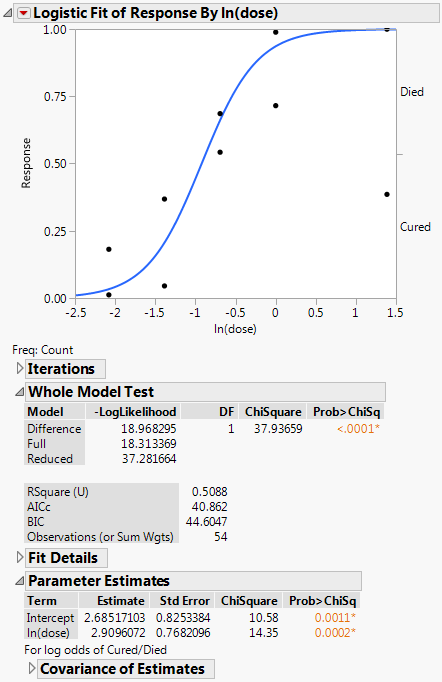Basic Analysis > Logistic Analysis > Example of Nominal Logistic Regression
Publication date: 08/13/2020

# Example of Nominal Logistic Regression

This example uses the Penicillin.jmp sample data table. The data in this example comes from an experiment where 5 groups, each containing 12 rabbits, were injected with streptococcus bacteria. Once the rabbits were confirmed to have the bacteria in their system, they were given different doses of penicillin. You want to find out whether the natural log (In(dose)) of dosage amounts has any effect on whether the rabbits are cured.

1. Select Help > Sample Data Library and open Penicillin.jmp.

2. Select Analyze > Fit Y by X.

3. Select Response and click Y, Response.

4. Select ln(dose) and click X, Factor.

Notice that JMP automatically fills in Count for Freq. Count was previously assigned the role of Freq.

5. Click OK.

Figure 8.2 Example of Nominal Logistic ReportThe plot shows the fitted model as a function of ln(dose). The fitted model is the predicted probability of being cured. The p-value is significant, indicating that the dosage amounts have a significant effect on whether the rabbits are cured.

Tip: To change the response level that is analyzed, specify a Target Level in the launch window or use the Value Order column property.